6419.0 - Producer and International Trade Price Indexes, 1995
Latest ISSUE Released at 11:30 AM (CANBERRA TIME) 13/02/1995  Ceased
Page tools:Print AllRSSSearch this Product
Contents >> Chapter 3. Basic features of price index construction

Elements and formulae

Common elements found in price indexes

3.1. All Producer and International Trade Price Indexes have the following elements:

• A regimen. A list of index items selected to represent the range of goods within the scope of the particular index. The regimen for the Import Price Index, for example, is representative of goods imported into Australia.
• Weights. The proportions in which price changes are combined are called weights and represent the relative importance of each index item in the total index.
• Prices. The prices of a selection of specific goods to represent each item in the regimen.
• A reference base. This is the base period of the index and is given a value of 100.0 (or 100). This value has been chosen as the base figure because it enables the ready calculation of percentage changes from the base period to the current period (i.e. current index number less 100 gives the percentage change from the base period).

Laspeyres formula

3.2. All the indexes are compiled using the Laspeyres fixed weight formula: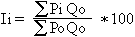where: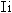represents index numbers for the period i;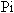represents prices in period i;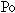represents base period prices; and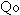represents base period quantities.Previous Page Next Page